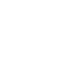Question searchedTop matches
Q1.
Two circles of radii 5 cm and 3 cm intersect at two points and the distance between their centres is 4 cm. The length of common chord   is
Q2.

Two circles of radii 5 cm and 3 cm intersect at two points and the distance between their centers is 4 cm. Find the length of the common chord.

Q3.
Two circle of radii 5 cm and 3 cm intersect at two points and the distance between their centres is 4 cm. The length of their common chord  is
Q4.

Two circles of radii 4 cm and 3 cm intersect at two points and the distance between their centres is 5 cm. Find the length of the common chord.

Q5.
Two circles of radii 10 cm and 8 cm intersect at two points and  the length of the common chord is 12 cm. Find the distance between their centres.